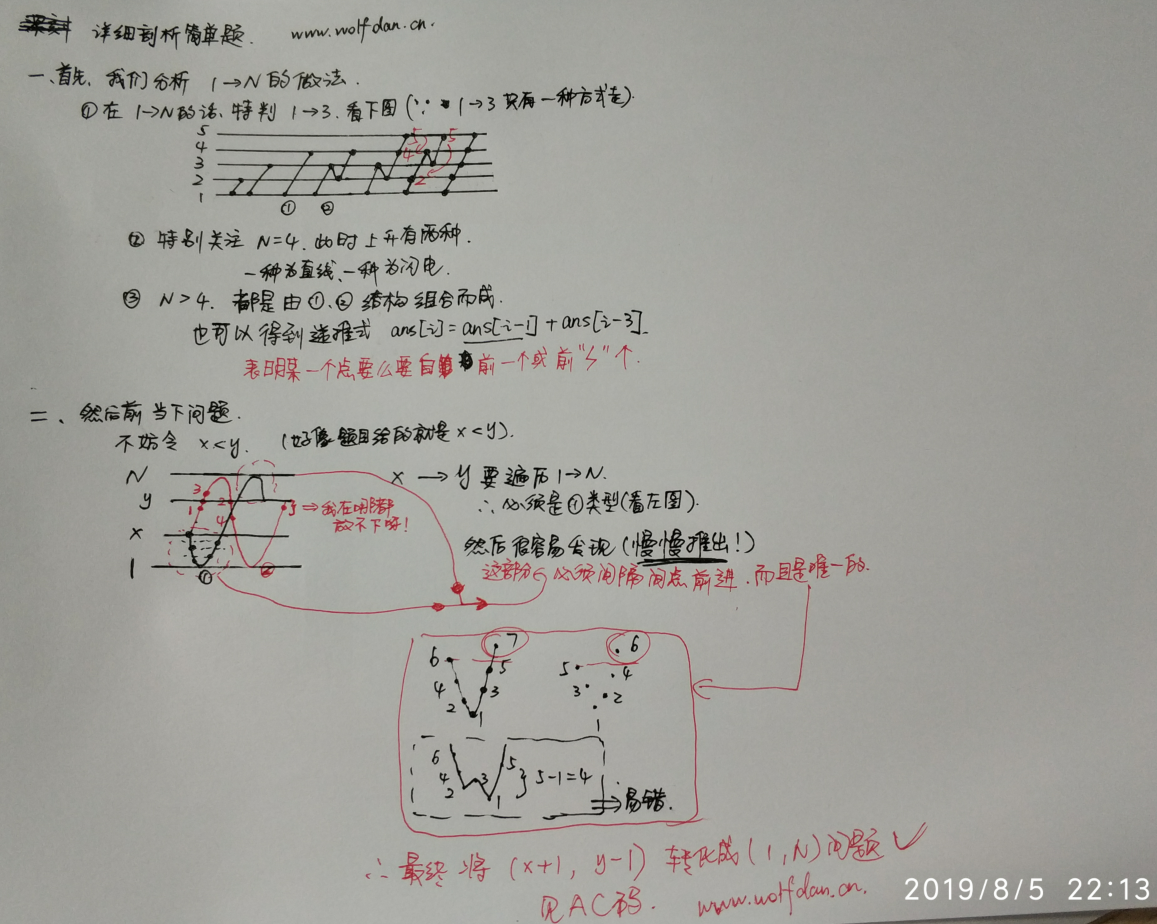2019杭电多校1006和1007算法日常[4/100]

# 序

• 今天是杭电多校第5场，然后1006签到本来应该10mins内写完，然后我菜鸡写了2小时(各种问题不熟练紧张)，赛后发现有大佬用dc3(一种据说复杂度O(n)的后缀数组算法,发现自己孤陋寡闻)
• 1007真滴有趣…闪电蛇皮走位，然后自己想复杂了一点点…

# 题目

2019杭电多校5

## 1006解法

Ekmp，用s.substr(1)的串来做ekmp函数的原串，s做ekmp函数的匹配串，这样跑一次ekmp就行，然后累加extend

ekmp学习教程·我觉得比较好的一个(我也用这个学的)

## AC代码

#include <iostream>
#include <string>
using namespace std;
long long ans;
// int next;
int nxt;
int extend;
string S, T;
int n, m;
/* 求解 T 中 next[]，注释参考 GetExtend() */
void GetNext(string & T, int & m, int next[])
{
int a = 0, p = 0;
next = m;

for (int i = 1; i < m; i++)
{
if (i >= p || i + next[i - a] >= p)
{
if (i >= p)
p = i;

while (p < m && T[p] == T[p - i])
p++;

next[i] = p - i;
a = i;
}
else
next[i] = next[i - a];
}
}

/* 求解 extend[] */
void GetExtend(string & S, int & n, string & T, int & m, int extend[], int next[])
{
int a = 0, p = 0;
GetNext(T, m, next);

for (int i = 0; i < n; i++)
{
if (i >= p || i + next[i - a] >= p) // i >= p 的作用：举个典型例子，S 和 T 无一字符相同
{
if (i >= p)
p = i;

while (p < n && p - i < m && S[p] == T[p - i])
p++;

extend[i] = p - i;
a = i;
}
else
extend[i] = next[i - a];
}
}

int main(){
ios::sync_with_stdio(false); cin.tie(0);
int t;
cin>>t;
while(t--){
ans = 0;
cin>>S;
n = S.size();
T = S.substr(1);
m = n - 1;
/*可能程序以为我用了前面的函数声明中的next[],所以说我模棱两可,加个全局的命名空间就行
或者换个变量名也行*/
// GetExtend(T, m, S, n, extend, ::next);
GetExtend(T, m, S, n, extend, nxt);
for (int j = 0; j < m; j++){
// cout << extend[j] << " \n"[j==m-1];
ans += extend[j]+j==n-1 ? extend[j] : extend[j]+1 ;
}

cout << ans << endl;
}
return 0;
}


## 1007解法

### 法一

a[i] = a[i-1]+a[i-3],就是对于第i项有两种情况，要么是直接往上走要么是闪电### 法二

dls说暴力打表找规律比较不用动脑子，哭了，臭大佬，好过分

## AC代码

#include <bits/stdc++.h>
using namespace std;
typedef long long ll;
const ll mod = 998244353;
const int inf = 0x3f3f3f3f;
const int maxn = 100000 + 5;

ll a[maxn];

int main() {
a = a = a = 1;
for(int i = 4; i < maxn; i++) a[i] = (a[i-1]+a[i-3])%mod;
int T; scanf("%d", &T);
while(T--) {
int n, l, r;
scanf("%d%d%d", &n, &l, &r);
if(l > r) swap(l, r);
if(l != 1) l++;
if(r != n) r--;
printf("%lld\n", a[r-l+1]);
}

return 0;
}


# 每天一句叨叨(No Ratings Yet)Loading...